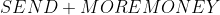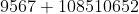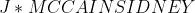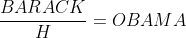0

## US Presidential Candidate Puzzles

Published on Thursday, July 24, 2008 in , ,Due to yesterday's surprise Mental Gym outage, I didn't have as much time as I'd like to prepare today's column. Instead, I'd like to post two original puzzles I've been saving.

These puzzles are both cryptarithms. Cryptarithms, for those who aren't familiar with them, are puzzles in which you're given a mathematical expression where all the digits, 0 through 9, are replaced by letters, with a given digit always being replaced by the same letter, and a given letter representing only one particular digit. Probably the most famous puzzle of this type is Henry Dudeney's classic from the July 1924 issue of Strand Magazine:The unique answer to this problem is:Note that the D has been replaced by a 7, the Es have been replaced by 5s, the Ms by 1s, the N by 6, the Os by 0s (hey, that's handy!), the R by 8, the S by 9, and the Y by 2.

As you can see, you have to look at how each letter interacts with the others in the mathematical expression to work out which digit is represented by which letter.

The following two cryptarithms I am going to give you, both of which are original with me, concern the US presidential candidates from both of the major parties in the 2008 election.

In both cases, these rules are in effect:

• Any given letter always represents only one corresponding digit (0-9).
• Any given digit (0-9) is always represented by only one corresponding letter.
• The leftmost digit of any number is never 0 (For example, in SEND + MORE = MONEY, neither the S nor the Ms are allowed to represent 0).
• When all the letters are replaced by their corresponding digits, the resulting mathematical equation must be correct.
• All numbers involved are whole numbers.
• Each puzzle has only one solution.The second puzzle concerns the Democrat presidential candidate, Barack Obama:I'm not going to give the answers now. If no one solves these by the time I post on Sunday, July 27th, I'll include the answers at the end of my next entry.